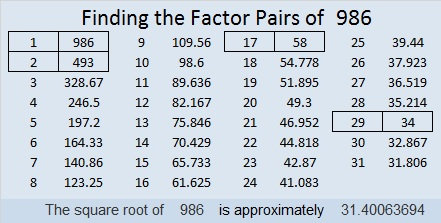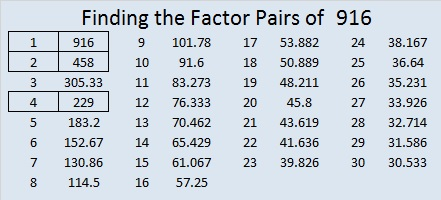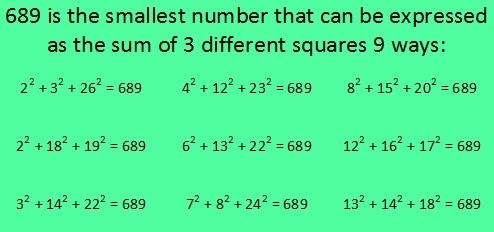# 986 and Level 1

Today’s puzzle looks like a simple holiday napkin, and it’s actually quite simple to solve. Even kids who have learned how to multiply but haven’t even heard of division could solve this puzzle.Print the puzzles or type the solution in this excel file: 10-factors-986-992

986 looks the same upside-down as it does right-side up, so it is a strobogrammatic number.

31² + 5² = 986
25² + 19² = 986

986 is the hypotenuse of FOUR Pythagorean triples:
264-950-986
310-936-986
680-714-986
464-870-986

986 looks interesting in some other bases:
12421 BASE 5
TT in BASE 33 (T is 29 base 10)
T0 in BASE 34

• 986 is a composite number.
• Prime factorization: 986 = 2 × 17 × 29
• The exponents in the prime factorization are 1, 1, and 1. Adding one to each and multiplying we get (1 + 1)(1 + 1)(1 + 1) = 2 × 2 × 2 = 8. Therefore 986 has exactly 8 factors.
• Factors of 986: 1, 2, 17, 29, 34, 58, 493, 986
• Factor pairs: 986 = 1 × 986, 2 × 493, 17 × 58, or 29 × 34
• 986 has no square factors that allow its square root to be simplified. √986 ≈ 31.4006# 916 Witch’s Hat

Today’s puzzle looks a little like a witch’s hat. Solving it could be bewitching. It contains a lot of perfect squares, and it’s only a level 2. Give it a try! Just make sure you don’t write a number greater than ten in the top row or the first column. Use all the numbers from one to ten in both places. There is only one solution.Print the puzzles or type the solution on this excel file: 10-factors-914-922

916 looks the same upside-down as it does right-side up, so it is a Strobogrammatic number.It looks like someone put a spell on that number!

Not only that, in BASE 26, our 916 looks like 196. There is a whole lot of magic going on here!

30² + 4² = 916, so 916 is the hypotenuse of a Pythagorean triple:
240-884-916, which can be calculated from 2(30)(4), 30² – 4², 30² + 4².

• 916 is a composite number.
• Prime factorization: 916 = 2 × 2 × 229, which can be written 916 = 2² × 229
• The exponents in the prime factorization are 2 and 1. Adding one to each and multiplying we get (2 + 1)(1 + 1) = 3 × 2  = 6. Therefore 916 has exactly 6 factors.
• Factors of 916: 1, 2, 4, 229, 458, 916
• Factor pairs: 916 = 1 × 916, 2 × 458, or 4 × 229
• Taking the factor pair with the largest square number factor, we get √916 = (√4)(√229) = 2√229 ≈ 30.2654919# What Makes 689 Amazing?

689 is an amazing number for several reasons. I decided to make graphics to illustrate many of those ways. (689’s factoring information is at the end of this post.)

689 is the sum of consecutive prime numbers 227, 229, and 233.

Also 689 is the sum of the primes from 83 to 109. Do you know what those 7 prime numbers are?

OEIS.org informs us that 689 is the smallest number that can be expressed as the sum of three different square numbers NINE ways. I decided to figure out what those nine ways are and make this first graphic to share with you:Note: 614 can also be expressed as the sum of 3 squares 9 different ways, but one of those ways is 17² + 17² + 6² = 614, and that duplicates 17² in the same sum.

689 is the same number when it is turned upside down. Numbers with that characteristic are called Strobogrammatic numbers.689 BASE 10 isn’t a palindrome, but 373 BASE 14 is; note that 3(196) + 7(14) + 3(1) = 689

Both of 689’s prime factors have a remainder of 1 when divided by 4, so they are hypotenuses of Pythagorean triples. That fact also means 689 can be expressed as the sum of two square numbers TWO different ways, and it makes 689 the hypotenuse of FOUR Pythagorean triples.  Can you tell by looking at the graphic which two are primitive and which two aren’t?689 is the sum of consecutive numbers three different ways:

• 344 + 345 = 689; that’s 2 consecutive numbers.
• 47 + 48 + 49 + 50 + 51 + 52 + 53 + 54 + 55 + 56 + 57 + 58 + 59 = 689; that’s 13 consecutive numbers.
• 14 + 15 + 16 + 17 + 18 + 19 + 20 + 21 + 22 + 23 + 24 + 25 + 26 + 27 + 28 + 29 + 30 + 31 + 32 + 33 + 34 + 35 + 36 + 37 + 38 + 39 = 689; that’s 26 consecutive numbers.

Now you have a few reasons why 689 is an amazing number. 13 and 53 were part of some of those reasons so it shouldn’t surprise anyone to see 13 and 53 pop up in its factoring information, too:

——————————————————————————

• 689 is a composite number.
• Prime factorization: 689 = 13 x 53
• The exponents in the prime factorization are 1 and 1. Adding one to each and multiplying we get (1 + 1)(1 + 1) = 2 x 2 = 4. Therefore 689 has exactly 4 factors.
• Factors of 689: 1, 13, 53, 689
• Factor pairs: 689 = 1 x 689 or 13 x 53
• 689 has no square factors that allow its square root to be simplified. √689 ≈ 26.248809.